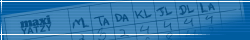Highscore Top 20
...The Top 100 list...Last months no. 1
600p - Peter-BuaTop 20 this month
...this months Top 100...Top 20 todayTo be able to play 3d maxi yahtzee dice game online and get on the high score list you need to install Java!

Maxi Yahtzee rules:
Three rolls in each turn. One roll must be made in each turn. Unused rolls are saved to the following turns.PointsExample Ones The number of eyes on the dice that show 1. 3 1 1 6 1 4=3 points Twos The number of eyes on the dice that show 2. 2 5 4 3 2 6=4 points Threes The number of eyes on the dice that show 3. 3 2 1 3 4 3=9 points Fours The number of eyes on the dice that show 4. 4 4 3 4 5 4=16 points Fives The number of eyes on the dice that show 5. 5 1 2 5 5 4=15 points Sixes The number of eyes on the dice that show 6. 4 6 3 1 6 6=18 points Bonus 100 points if the sum of the fields Ones to Sixes is 84 points or more. If the sum is 83 points or less then bonus is 0 points. One pair Two dice with the same value. The number of eyes on the two dice are counted. 6 4 2 6 5 1=12 points Two pairs Two pairs. The pairs have to have different values. 2 1 3 3 5 5=16 points Three pairs Three pairs. The pairs have to have different values. 2 2 5 5 6 6=26 points 3 of a kind Three dice with the same value. 4 5 3 5 5 4=15 points 4 of a kind Four dice with the same value. 2 4 2 2 5 2=8 points 5 of a kind Five dice with the same value. 3 3 2 3 3 3=15 points Small straight The dice have to show 1 2 3 4 5. The last die can show anything. Small straight is worth 15 points. 1 2 3 4 5 3=15 points Large straight The dice have to show 2 3 4 5 6. The last die can show anything. Large straight is worth 20 points. 2 3 4 5 6 6=20 points Full straight The dice have to show 1 2 3 4 5 6. Full straight is worth 21 points. 1 2 3 4 5 6=21 points Full house 2+3 One pair and '3 of a kind'. The dice in the pair and in the '3 of a kind' have to have different values. 3 4 4 6 6 6=26 points Villa 3+3 Two '3 of a kind'. The dice in the '3 of a kind' have to have different values. 2 2 2 5 5 5=21 points Tower 2+4 One pair and '4 of a kind'. The dice in the pair and in the '4 of a kind' have to have different values. 3 3 6 6 6 6=30 points Chance Count all the eyes on the dice. 3 4 2 6 5 4=24 points Maxi yahtzee All the dice have to have the same value. Maxi Yahtzee is always worth 100 points. 4 4 4 4 4 4=100 points